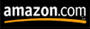A mathematical proof which explains why managers and others in authority make so much money.  From your high school physics, you will recall
that:

Work
Power  =  -------
Time

>From the world of business, we know that:

Knowledge =  Power

and also that:

Time  = Money,

Substituting these identities into the original equation, we get:

Work
Knowledge =   --------
Money

Solving for money, we get:

Work
Money =     ------------
Knowledge

Thus, Money approaches infinity as Knowledge approaches zero, regardless of the Work done.  What this means is:  The Less you Know, the More you Make.

Taking this analysis and proof one step further, we know that:

Knowledge =  Education x Time

Solving for Time:

Knowledge
Time   =   ------------
Education

and then substituting for Time back into the Power equation, we get:

Work         Work  x Education
Power   =  -------    =   -------------------

Time         Knowledge

>From which we can see that the closer Knowledge gets to 0, the more
power one will have.  Note:  You can also increase Power through increasing either Work or Education, but it should be noted that this approach doesn't have the same type of leverage as lack of knowledge.

This equation is also known as the MANAGEMENT Equation, since it provides a clear and concise mathematical explanation of why managers, executives, politicians and most other highly unknowledgeable individuals get paid so much.